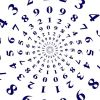#### You may also like### Curvy Equation

This problem asks you to use your curve sketching knowledge to find all the solutions to an equation.### Digital Equation

Can you find a three digit number which is equal to the sum of the hundreds digit, the square of the tens digit and the cube of the units digit?### Euler's Totient Function

How many numbers are there less than $n$ which have no common factors with $n$?

# Square Difference

##### Age 16 to 18Challenge Level

All numbers referred to in this question are non-negative integers.

1. Express each of the numbers $3, 5, 8, 12$ and $16$ as the difference of two non-zero squares.
Can you notice any patterns in your answers? You could try a few more numbers as well. Are there any numbers that you cannot write as a difference of two squares?

2. Prove that any odd number can be written as the difference of two squares.
Have a look at your expressions for odd numbers in part (1), and try to express a few more odd numbers as a difference of two squares.  Can you find a pattern that they all follow?  Be careful - some numbers such as $21$ can be written as a difference of two squares in more than one way, and some ways won't fit the same pattern as the others.
Can you use algebra, or a diagram, to show that every odd number can be written as a difference of two squares (in at least one way!).

3. Prove that all numbers of the form $4k$, where $k$ is a non-negative integer, can be written as the difference of two squares.
If a number can be written in the form $4k$, then it is a multiple of $4$, i.e. $4, 8, 12, ...$
Again, look at patterns from your answers for $8, 12$ and $16$.  Can you also express $20$ and $24$ as a difference of two squares?  If we allow zero squares (which is ok in this part!) then we have $4=2^2-0^2$. Can you spot a pattern and use algebra or a diagram to prove that every number which is a multiple of $4$ can be written as difference of two squares?

4. Prove that no number of the form $4k+2$, where $k$ is a non-negative integer, can be written as the difference of two squares.
If a number has the form $4k+2$ then it is even, but it is not a multiple of $4$.  For example we have $6=4\times 1 + 2$ and $34 = 4 \times 8 + 2$.
For this part you need to show that no matter what you do, you cannot make a number of the form $4k+2$ from a difference of two squares. You could start by considering the parity of a number of the form $A^2-B^2$, according to the different parities of $A$ and $B$.

*Considering the parity of a number means looking at whether it is odd or even.

5. Prove that any number of the form $pq$, where $p$ and $q$ are prime numbers greater than $2$, can be written as the difference of two squares in exactly two distinct ways. Does this result hold if $p$ is a prime greater than $2$ and $q=2$?
Examples might be helpful again.  A good starting point is to consider $p=3$, $q=5$.  Can you write $15$ as the difference of two squares in two different ways?  It might be helpful to look back to your answer to part (1) for inspiration for one of the ways.  You could also look at other examples.

Can you use your examples to make generalisations to prove that a number of the form $pq$, where $p$ and $q$ are primes greater than $2$, can be written as difference of two squares in two different ways.

The question asks you to show that it can be written as a difference of two squares in exactly two ways - can you show that there are no other ways of doing it?

If we have $pq=a^2-b^2$, can you use the difference of two squares formula to help you?

6. Determine the number of distinct ways in which $675$ can be written as the difference of two squares.
A good place to start is by writing $675$ as a product of prime factors.  You might also want to include a factor of $1$.

STEP Mathematics I, 2014, Q1. Question reproduced by kind permission of Cambridge Assessment Group Archives. The question remains Copyright University of Cambridge Local Examinations Syndicate ("UCLES"), All rights reserved.